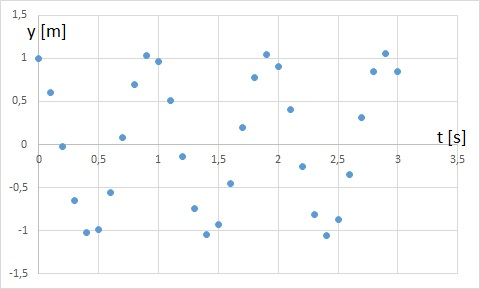# Simple harmonic motion –Euler’s step method

Up a level : Differential Equations
Previous page : Euler’s step method
Next page : The Earth orbit and Euler’s Step methodOk, let’s look at$y'' = - \frac{k}{m}y$

one more time. In this case we need to calculate the acceleration (y´´) given the displacement, then the change of velocity and the velocity (y´) given the acceleration, and finally a new position given the velocity. I.e.$\begin{gathered} a = - \frac{k}{m}y, \hfill \\ v \leftarrow v + a\Delta t, \hfill \\ y \leftarrow y + v\Delta t \hfill \\ \end{gathered}$

Then the above is repeated over and over again. The arrows stands for “is given by” and is used so that we don’t need to create a new variable name for each new position.

To test this we choose our k and m so that the period will be 1 s. According to the previously found solution we have that$\omega = \sqrt {\frac{k}{m}} = 2\pi f$

so$T = 2\pi \sqrt {\frac{m}{k}}$

For T=1 s we get${\left( {\frac{1}{{2\pi }}} \right)^2} = \frac{m}{k}$

So if we select k=10 N/m then$m = 10{\left( {\frac{1}{{2\pi }}} \right)^2} \approx 0.2530{\text{ kg}}$

The below is done in Excel with a time step size of 0.1 s and an initial amplitude of 1 m and using the above mass.

The below is done in Excel with a time step size of 0.1 s and an initial amplitude of 1 m and using the above mass. This is the contents of the first few cells:

 D E F G 3 g 9,81 4 k 10 5 m 0,253302959 6 dt 0,1 7 8 t y v a 9 0 1 0 =-$F$4/$F$5*E9 10 =D9+$F$6 =E9+F10*$F$6 =F9+G9*$F$6 =-$F$4/$F$5*E10

Then row 10 is simply copied down a few hundred cell. This is the result for the first 3 s.

 t y v a 0.0 1.0000 0.0000 -39.4784 0.1 0.6052 -3.9478 -23.8930 0.2 -0.0285 -6.3371 1.1251 0.3 -0.6510 -6.2246 25.6989 0.4 -1.0164 -3.6547 40.1273 0.5 -0.9806 0.3580 38.7140 0.6 -0.5577 4.2294 22.0170 0.7 0.0854 6.4311 -3.3719 0.8 0.6948 6.0939 -27.4296 0.9 1.0299 3.3509 -40.6586 1.0 0.9584 -0.7149 -37.8362 1.1 0.5085 -4.4985 -20.0767 1.2 -0.1421 -6.5062 5.6088 1.3 -0.7366 -5.9453 29.0800 1.4 -1.0403 -3.0373 41.0709 1.5 -0.9334 1.0698 36.8476 1.6 -0.4579 4.7545 18.0775 1.7 0.1983 6.5623 -7.8293 1.8 0.7763 5.7793 -30.6453 1.9 1.0477 2.7148 -41.3630 2.0 0.9056 -1.4215 -35.7512 2.1 0.4059 -4.9966 -16.0254 2.2 -0.2540 -6.5991 10.0269 2.3 -0.8136 -5.5964 32.1208 2.4 -1.0521 -2.3844 41.5339 2.5 -0.8752 1.7690 34.5501 2.6 -0.3528 5.2240 13.9264 2.7 0.3089 6.6167 -12.1952 2.8 0.8486 5.3972 -33.5023 2.9 1.0533 2.0469 -41.5832 3.0 0.8422 -2.1114 -33.2478

The Excel file for this can be found here: Simple harmonic motion. It uses decimal comma, so you might have to change that to decimal point in the cells for the constants.

The figure below shows the same in a graphical form. As you can see the period is slightly shorter than 1 s.With a smaller step size, 0,001 we get the following graph. It is now very close to a sine wave with amplitude 1 and period 1. Matter a fact at 1 s the y value is about 0.99997.Up a level : Differential Equations
Previous page : Euler’s step method
Next page : The Earth orbit and Euler’s Step methodLast modified: Mar 31, 2019 @ 12:28